## Hyperbolic Sine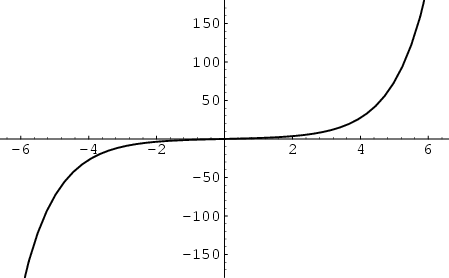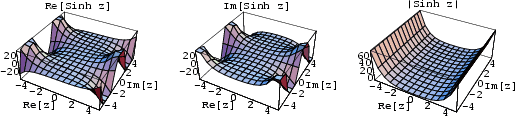The hyperbolic sine is defined as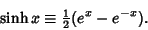See also Beta Function (Exponential), Bipolar Coordinates, Bipolar Cylindrical Coordinates, Bispherical Coordinates, Catenary, Catenoid, Conical Function, Cubic Equation, de Moivre's Identity, Dixon-Ferrar Formula, Elliptic Cylindrical Coordinates, Elsasser Function, Fibonacci Hyperbolic Cosine, Fibonacci Hyperbolic Sine, Gudermannian Function, Helicoid, Helmholtz Differential Equation--Elliptic Cylindrical Coordinates, Hyperbolic Cosecant, Laplace's Equation--Bispherical Coordinates, Laplace's Equation--Toroidal Coordinates, Lebesgue Constants (Fourier Series), Lorentz Group, Mercator Projection, Miller Cylindrical Projection, Modified Bessel Function of the Second Kind, Modified Spherical Bessel Function, Modified Struve Function, Nicholson's Formula, Oblate Spheroidal Coordinates, Parabola Involute, Partition Function P, Poinsot's Spirals, Prolate Spheroidal Coordinates, Ramanujan's Tau Function, Schläfli's Formula, Shi, Sine, Sine-Gordon Equation, Surface of Revolution, Toroidal Coordinates, Toroidal Function, Tractrix, Watson's Formula

References

Abramowitz, M. and Stegun, C. A. (Eds.). Hyperbolic Functions.'' §4.5 in Handbook of Mathematical Functions with Formulas, Graphs, and Mathematical Tables, 9th printing. New York: Dover, pp. 83-86, 1972.

Spanier, J. and Oldham, K. B. The Hyperbolic Sine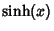and Cosine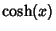Functions.'' Ch. 28 in An Atlas of Functions. Washington, DC: Hemisphere, pp. 263-271, 1987.